7 mins

Edit

# 3d modeling as a developer

As a developer, 3d modeling was something that I had always wanted to try since it was something that I needed to visualize the final product or to create prototypes in my DIY projects. For a long time I’ve been searching for a perfect CAD, but none of them were ideal for me. During one of my talks with a colleague of mine, he mentioned something about TinkerCAD, Fusion360 and OpenSCAD. The last one immediately got my attention as it had “open” in its name. I asked him to elaborate on that but “that’s geeky stuff” was all that he said. I’ve decided then to look it up since I had a feeling that it might be something for me…

OpenSCAD is a small (approx. 25 MB) but powerful CAD that lets us design using it’s own language. Its design is very simple and splits into 3 areas.

1. Editor
2. Preview
3. Console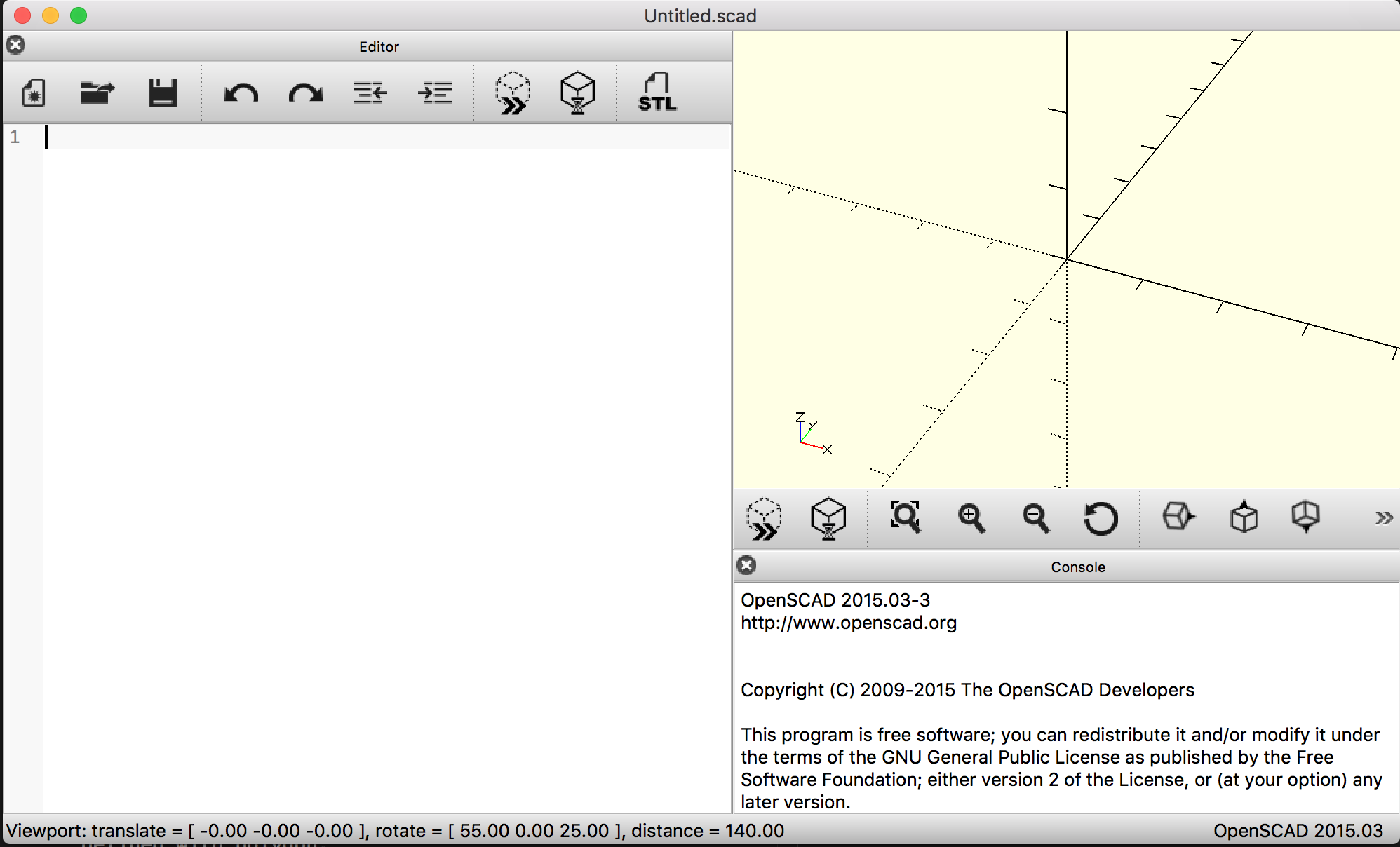The best in openSCAD is its simplicity. All you need to know are a few shapes and functions, and that’s all. This cheat sheet might come in handy as all the important knowledge is included in it.

# How it works

Nearly everything can be created with functions. Syntax tree looks mostly like this:

``````object();
variable = value;
operator() action();
operator() {
action();
action();
}
operator() operator() {
action();
action();
}
operator() {
operator() action();
operator() {
action();
action();
}
}
``````

For example, if we want to create a cylinder, we just have to call `cylinder(h,r|d,center)`:

• `h` is height
• `r` is radius
• `d` is diameter
``````cylinder(h=2, r=5);
``````

`center` parameter is not obligatory. It moves center of our shape to [0,0,0].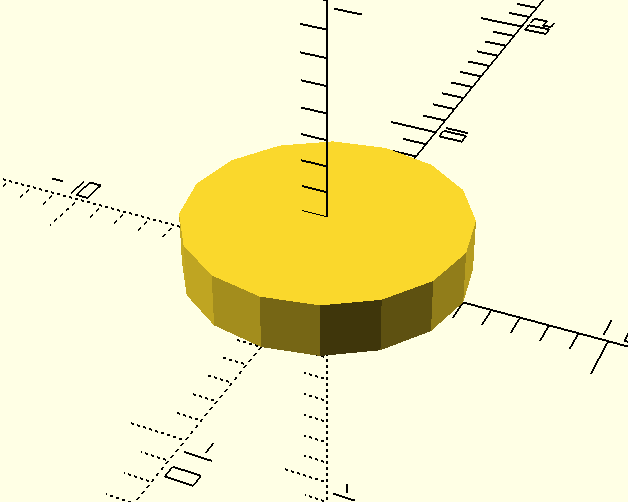You should name all the parameters. If you don’t, it’s more likely that you will make a mistake. If you called a previous function with two parameters but without names: `cylinder(2, 5);`, the result would be following: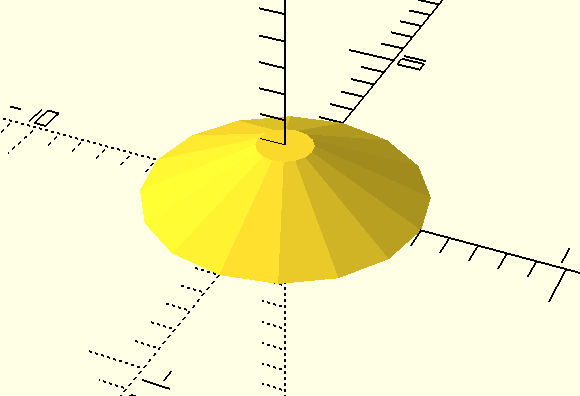So what is happening?

Function without the named parameters will be called like `cylinder(h = 2, r1 = 5, r2 = 1);` Because `r2` is `1` by default when `r1` is defined. You can read more about this here.

# Operations on shapes

With the help of documentation we can create 3d shapes like spheres, cubes etc. But what if we’d like to create some more complicated figures? We need to wrap our figures with operation functions:

• `union` - sum
• `difference` - subtraction
• `intersection` - overlapping part of shapes

So if you want to create crossed cubes, below you can find the way how you can do this:

``````union() {
cube(size=[2,2,10], center=true); //first cube
cube(size=[2,10,2], center=true); //second cube
}
``````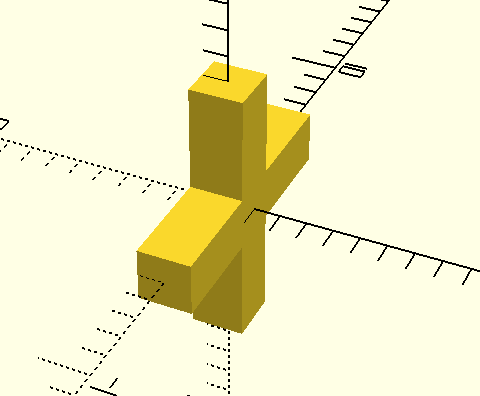Cube with gap in the middle:

``````difference() {
cube(size=[2,2,10], center=true); //first cube (base)
cube(size=[2,10,2], center=true); //second cube (will be subtracted)
//nth shape will be also subtracted from base
}
``````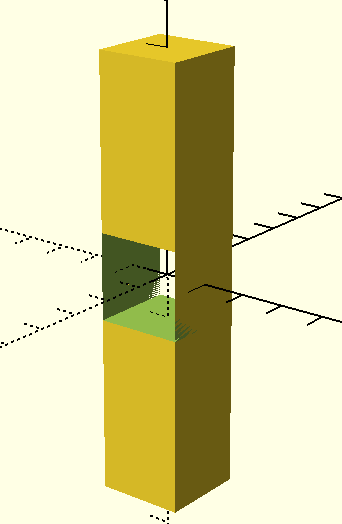And… we’ve encountered a glitch here. We have a hole in the middle of our cube, but because the width of the first cube equals the width of the second cube, we have walls with width 0. In preview it may be a bit distracting, but if we render it (F6 or Design > Render) it should disappear. The second way is to make subtracted shape a bit wider.

``````difference() {
cube(size=[2,2,10], center=true); //first cube (base)
cube(size=[2.01,10,2], center=true); //second cube (will be subtracted)
//nth shape will be also subtracted from the base
}
``````

The common part of two cubes - should be cube with dimensions 2x2x2:

``````intersection() {
cube(size=[2,2,10], center=true);
cube(size=[2,10,2], center=true);
}
``````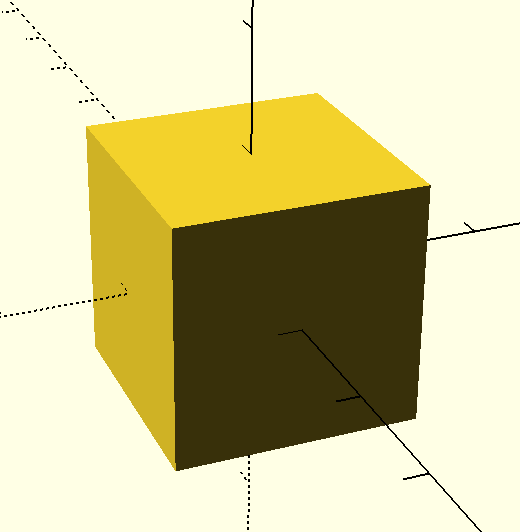It is possible to wrap operations in operations. For example, at first `rotate` cube and then subtract it from another one.

``````difference() {
cube(size=[2,2,10], center=true);
rotate([45,0,0]) cube(size=[2.01,10,2], center=true);
}
``````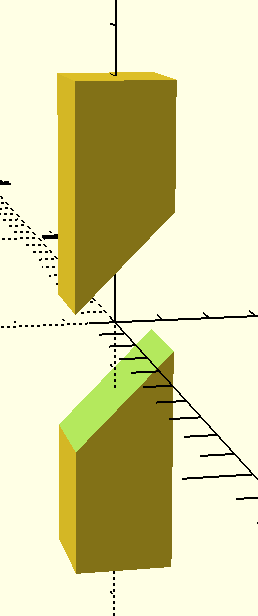There can be a useful debug function. Just put hash `#` before the operation:

``````difference() {
cube(size=[2,2,10], center=true);
#rotate([45,0,0]) cube(size=[2.01,10,2], center=true);
}
``````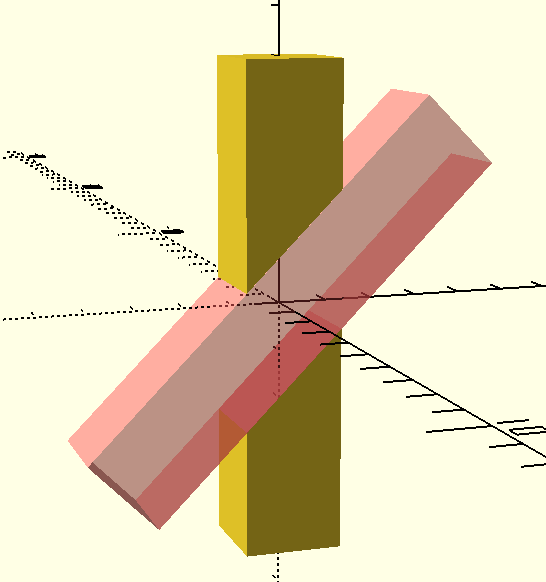Feel free to try other operations and transformations.

# Another basic useful stuff

## Conditional rendering

Sometimes we want to render something only in certain cases. OpenSCAD allows us to use `if` and it has a similar syntax to javascript etc.

``````a = 1;
if (a > 0) {
sphere(r=a);
}
``````

## For loop

Syntax: `for(variable = [start : increment : end]) {}` Let’s create holes around cylinder’s border.

``````radius=5;

difference() {

for(i=[0:360/6:360]) {
echo(i);
rotate([0,0,i])
cube(size=[1,1,1.1], center=true);
}
}
``````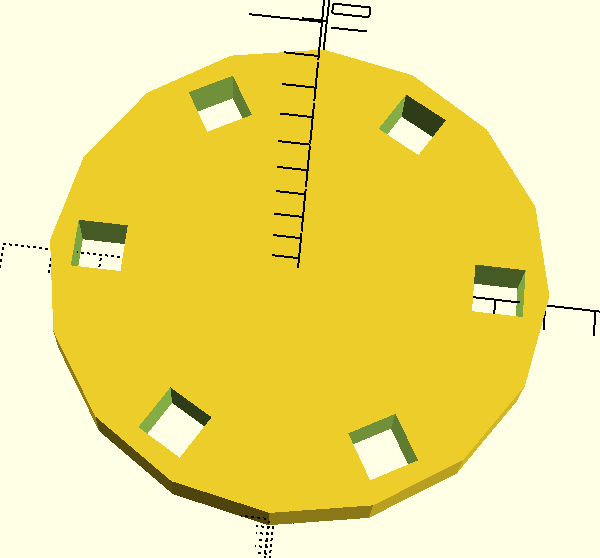## And others…

`echo` is useful for debugging. I often use also `import` for importing external `stl` files to the project. OpenSCAD has a lot of popular mathematical functions and transformations. It also allows us to create 2d models and extrude it to 3d models with `linear_extrude` or `rotate_extrude`. You can look for details here. Documentation is very easy to use so I believe this doesn’t need any further explaining.

# Last words

OpenSCAD is a very powerful tool if we know how to use it. I was able to create useable models in a few hours after reading the manual. If you are a developer and prefer writing a code to drawing, I think it will be perfect for you.